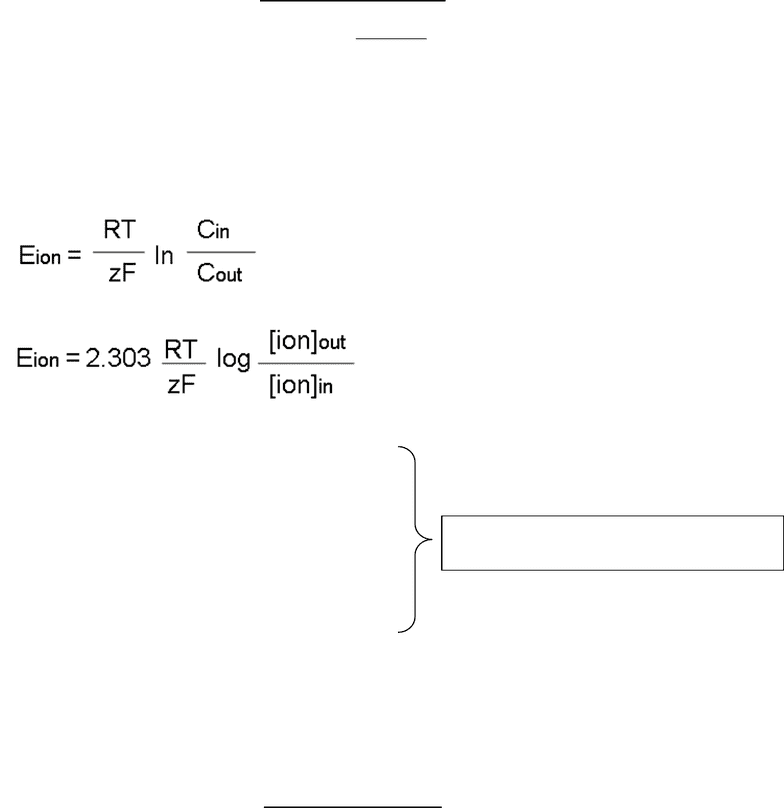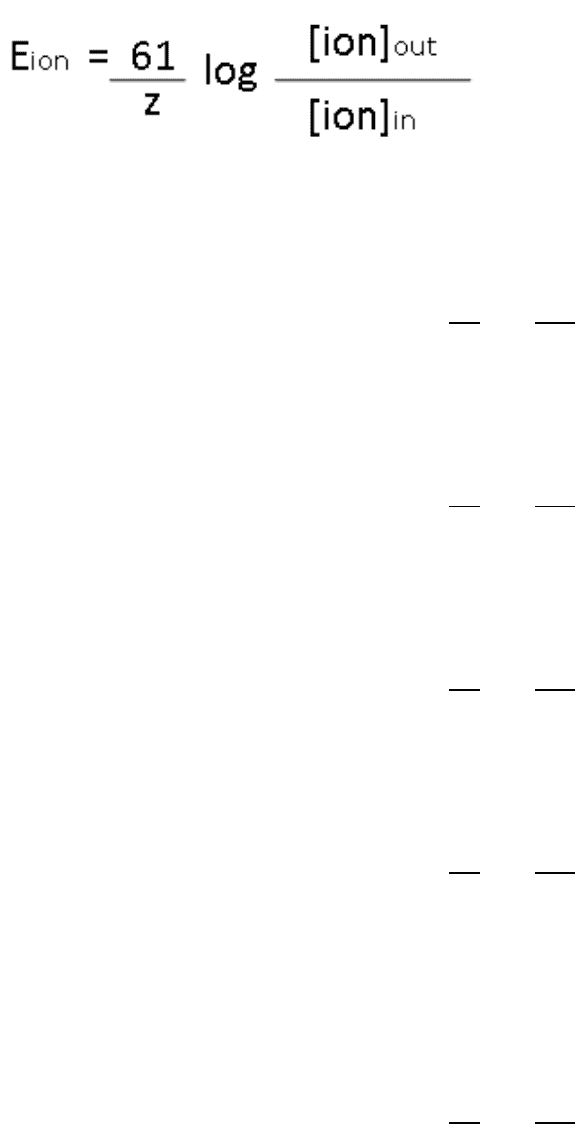Class Notes (1,100,000)
CA (620,000)
Brock U (10,000)
BIOL (1,000)
drfu (30)
Lecture 16

# BIOL 2P98 Lecture Notes - Lecture 16: Nernst Equation, Reversal Potential, Resting Potential

Department
Biological Sciences
Course Code
BIOL 2P98
Professor
drfu
Lecture
16

This preview shows page 1. to view the full 5 pages of the document.HLSC 2P09 1
Part 1: Nernst or Equilibrium Potential (for a single ion across the cell membrane):
See p.160-166 in Silverthorn textbook
The Nernst potential or Equilibrium potential of an ion is the potential across the membrane that
prevents net diffusion of the ion in either direction through the membrane.
The Nernst Equation is also known as the Equilibrium Potential or Eion and is as follows:
Eion = the equilibrium potential of an ion
R = is the universal gas constant (8.314 Jmol-1K-1)
T = temperature in Kelvin (K) at 310K (body temp)
Z = valence of the ion
F = Faradays constant (9.64853 x 104 coulombs/mole)
[ion]out is the ion concentration outside the cell
[ion]in is the ion concentration inside the cell
2.303 = conversion factor between ln & log
(𝟐. 𝟑𝟎𝟑)(𝟖. 𝟑𝟏𝟒)(𝟑𝟏𝟎)
(𝟗. 𝟔𝟒𝟖𝟓𝟑 𝒙 𝟏𝟎𝟒)
= 𝟎. 𝟎𝟔𝟏𝑽 𝒐𝒓 𝟔𝟏𝒎𝑽
The sum of 2.303RT/zF gives you a value of 61
###### You're Reading a Preview

Unlock to view full version

Only page 1 are available for preview. Some parts have been intentionally blurred.HLSC 2P09 2
In the text book the Nernst Equation is:
Practice Questions:
1) The [K+]in is 150mM and the [K+]out is 5mM, what is the equilibrium potential of K+ (EK+)?
𝐸 𝑖𝑜𝑛 = (61
+1)log(5
150)
= 90.1 𝑚𝑉
2) [Na+]out is 150mM and [Na+]in is 15mM. What is the equilibrium potential for Na+?
𝐸 𝑖𝑜𝑛 = (61
+1)log(150
15 )
= +61 𝑚𝑉
3) [Cl-]out is 145mM and [Cl-]in is 20mM. What is the equilibrium potential for Cl- ?
𝐸 𝑖𝑜𝑛 = (61
−1)log(145
20 )
= 52.5 𝑚𝑉
4) [K+]in is 250mM and [K+]out is 15mM. Calculate the Nernst potential for K+.
𝐸 𝑖𝑜𝑛 = (61
+1)log(15
250)
= 74.5 𝑚𝑉
5) In a squid axon, the internal potassium concentration is typically 400mM, and the external
potassium concentration in squid tissue is 20mM (these results are quite different from those for
man!). Calculate the Nernst equilibrium potential for potassium in a squid axon.
𝐸 𝑖𝑜𝑛 = (61
+1)log(20
400)
= 79.4 𝑚𝑉
###### You're Reading a Preview

Unlock to view full version